top of page

# GSA, MCRT, SRT, and Sludge Age

Updated: Sep 27, 2021

### GSA, MCRT, SRT, and Sludge Age Defined

I think there is confusion, sometimes, in how operators (which includes me) define the terms mean cell residence time (MCRT), solids retention time (SRT), sludge age, and, though seldom used these days (was it ever used?), Gould sludge age (GSA). In this post I want to have a detailed discussion about MCRT and SRT, leaving sludge age as a phrase that can be used to describe either of these two parameters equally well or as you may prefer.Often, and I have been guilty of this myself, mean cell residence time, solids retention time, and sludge age are used interchangeably. For this to be true these parameters would have to be calculated in exactly the same way and this is not truly the case with MCRT and SRT, though mathematically these two parameters are closely related. The difference between the formulas for MCRT and SRT is that the MCRT equation includes the solids in the secondary clarifier whereas the SRT calculation does not include clarifier solids, as shown graphically below.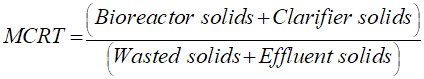Shortly, we'll go into the details of how these parameters are calculated but first up is a quick look at the Gould sludge age.

### Gould Sludge Age (GSA)

The Gould sludge age represents the average number of days total suspended solids (TSS) entering the bioreactor remain under aeration. The GSA is calculated by dividing the pounds (or kg) of MLSS in the aeration tank by the pounds (or kg) of total suspended solids entering the aeration tank as shown schematically in Figure No. 1.

The formula for calculating the GSA, which has units of days, appears below the schematic, in Equation No. 1. In truth, in more than 40 years, I have never once had a reason to calculate the GSA.

Figure No. 1: Gould Sludge AgeEquation No. 1: Formula for Gould Sludge Age (GSA)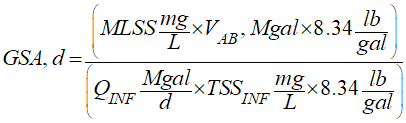### The 8.34 lb/gal Constant

In Equation No. 1 you can see the constant term "8.34 lb/gal" in both the numerator and the denominator. With these constant terms included you are seeing the "full" version of the equation, and these constants are required to correctly produce units of pounds for the MLSS in the bioreactor and the quantity of TSS pounds entering the bioreactor. But, because the constants are in both the numerator and denominator, they are redundant and can be cancelled out to simplify the equation, as shown in Equation No. 1A. For the rest of this post the equations will be shown with this constant removed, for the most part. Whether the constant is in a given equation, or cancelled out of the same equation, the result will be the same but the units in the numerator and denominator will no longer be "pounds of material."

Equation No. 1A: Formula for GSA with Constant Terms Removed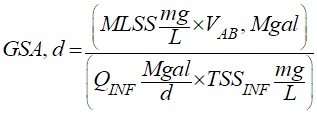And in case you are wondering, here is how the 8.34 lb/gal term is derived.### MCRT and SRT

Many operators refer to the mean cell residence time (MCRT), the solids retention time (SRT), and the sludge age as being one and the same and they'll use these three terms interchangeably. Because I am always going from one wastewater plant to another and I'm collecting data from these plants, I have to make sure I fully understand how the operators are actually defining these terms. So I always have to ask: "Are you including the solids in the secondary clarifier in your calculation of the MCRT/SRT/sludge age?" If the answer is YES, I then need to know how they are measuring and determining the solids in the secondary clarifier, because there are several ways this can be done, some ways perhaps being more accurate than other ways. I'll discuss this in more detail below. For now though, let me state my definition of the MCRT and the SRT, as I did previously, above.

MCRT (mean cell residence time) measures the mass of total suspended solids (TSS) in the treatment system, which includes the solids in the bioreactor (e.g., aeration basin) and the solids in the secondary clarifier, divided by the mass of TSS lost and removed from the treatment system. TSS is lost from the system as total suspended solids overflowing the secondary clarifier (plant effluent). TSS is removed from the system through the intentional wasting of activated sludge. The MCRT unit of measure is days.

SRT (solids retention time) measures only the mass of TSS (pounds of MLSS) contained in the bioreactor divided by the mass of TSS lost and removed from the treatment system. And just as with the MCRT calculation, TSS is lost from the system as solids overflowing the secondary clarifier (plant effluent TSS lb/d). TSS is removed intentionally from the system through the wasting of activated sludge (WAS lb/d). And also like the MCRT, the SRT unit of measure is days.

The simple process flow diagram in Figure No. 2 highlights where the pounds of material (solids) are coming from in the calculation of the MCRT and/or the SRT. Remember, the MCRT includes the solids in the secondary clarifier whereas the calculation of the SRT does not include the solids in the clarifier.

Figure No. 2: TSS Pounds In and Leaving An Activated Sludge System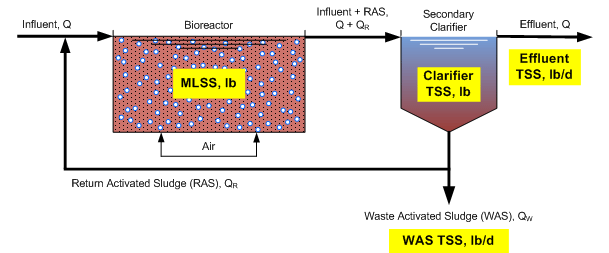### MCRT & SRT Equations

The formula for calculating the mean cell residence time (MCRT) is shown in Equation No. 2. Actually, one final time, I'm showing both versions of the MCRT equation, the first with the constants removed, the second with the constants included. The solids in the clarifier are included in the calculation.

The formula for calculating the solids retention time (SRT) is shown in Equation No. 3.

Equation No. 2: Formula for Mean Cell Residence Time (MCRT)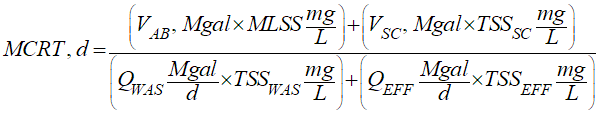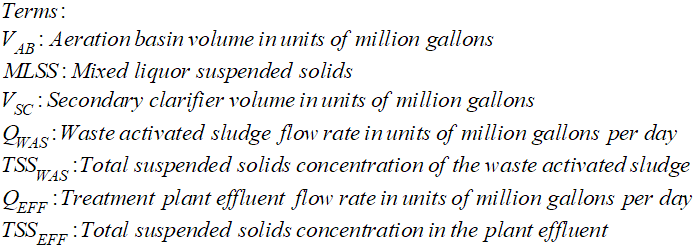Equation No. 3: Formula for Solids Retention Time (SRT)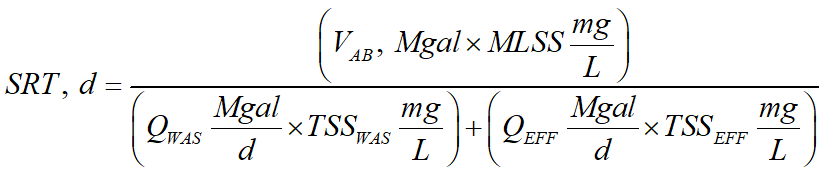### Difference Between MCRT and SRT

In Table No. 1 I shown an Excel spreadsheet using data from an industrial wastewater system where I have calculated both the MCRT and the SRT. As you can see, the SRT value is almost 32 percent lower than the MCRT value, the difference being the additional solids in the clarifier are increasing the MCRT value. And you might be thinking both the SRT and MCRT are on the high side but this is not uncommon for industrial wastewater systems treating complex organics. Longer SRT values may be required for the treatment of industrial wastewaters containing more difficult to degrade materials, which may also be inhibitory to biological growth (Grady, Daigger, Love, & Filipe, 2011).

Table No. 1: Comparison of MCRT and SRT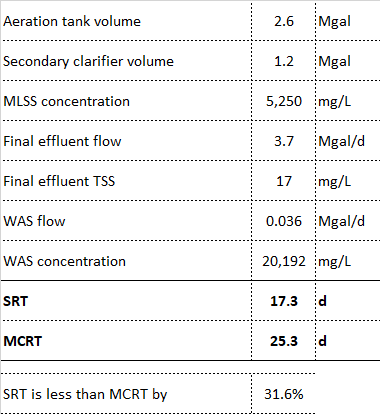In Table No. 1 the MCRT was calculated by using the MLSS concentration entering the secondary clarifier from the bioreactor. A full explanation of this approach is provided below, as described by "Method 4."

In Figure No. 3 you can see a distribution fit generated using @Risk (software program from Palisade) for 193 SRT values for an industrial wastewater system. The maximum SRT in the data set is 28 days, with 85% of the SRT values falling between 7 and 20 days.

Figure No. 3: @Risk Distribution Fit for SRT Data### Does It Matter Which Equation I Use?

Does it matter whether I use MCRT or SRT? NO!

Pick either equation and then use just that formula. My personal preference is to use the SRT because the MCRT requires determining the pounds of solids in the secondary clarifier. Doing this accurately is easier said than done, as I'll explain below.

### Why I Prefer SRT Over MCRT

Calculation of the SRT is easy because it uses data that is readily available and reliably produced. For the SRT the total suspended solids values you need are the MLSS, clarifier underflow TSS (the concentration in the return activated sludge or RAS) if you are wasting from the return sludge line, and the effluent TSS. When calculating the MCRT you need to know the solids in the secondary clarifier and how you make this determination can produce highly variable results.

Surprising to some, and confusing to many, is that there are four possible methods that can be used to calculate the MCRT with each method having to do with how the solids in the secondary clarifier are determined, as explained below.

1. The easiest, and perhaps clearest, method for calculating the MCRT is the one that does not include the solids in the secondary clarifier. The thinking behind taking this approach is that there should be a minimum quantity of solids in a well-operating clarifier. This approach is described in different textbooks such as in an excellent series of books from the Water Environment Federation called Operation of Municipal Wastewater Treatment Plants. But this method, per our entire discussion from above, means we are actually calculating the SRT, not the MCRT.

For me, it is easy to feel comfortable calculating the SRT, rather than the MCRT, without considering the clarifier solids. The clarifier is not intended to be a storage reservoir for solids. Rather, the goal of secondary clarification is to settle and return concentrated solids back to the bioreactor.

2. This method uses a clarifier core sample (sludge judge) to determine the total suspended solids in the column of water. You would obtain a sludge judge sample (or several) and discharge the volume into a container from which you would then measure the total suspended solids concentration. This is not difficult to do but it is time-consuming and, actually, a little aggravating to do, involving extra work that likely won’t be maintained and won't be performed consistently. I've done it and I don't like doing it!

3. Another method calculates the solids concentration in the clarifier as shown in Equation No. 4, taking into account the concentrations of the MLSS and the return activated sludge.

Equation No. 4: Clarifier Solids Concentration Formula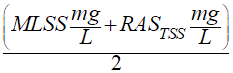4. The final method uses the MLSS concentration to calculate the solids in both the aeration basin (bioreactor) and the secondary clarifier. With this method you use the MLSS concentration and the total volume of the secondary clarifier to calculate the mass of solids in the system. Here’s how this works.

Look at the graphic in Figure No. 4. In Scenario A you have “steady-state conditions,” where mixed liquor suspended solids from the aeration basin are entering the secondary clarifier, settled sludge is being returned to the aeration basin (RAS or return activated sludge), waste activated sludge is being wasted from the RAS line, and clean, low-solids (low TSS), water is overflowing the clarifier. Even though Scenario A describes a dynamic operation with flow entering and leaving the clarifier, you still have solids concentrating (thickening) as you move vertically down to the bottom of the clarifier.

Figure No. 4: Calculating Pounds of MLSS in the Clarifier

Solids in the Clarifier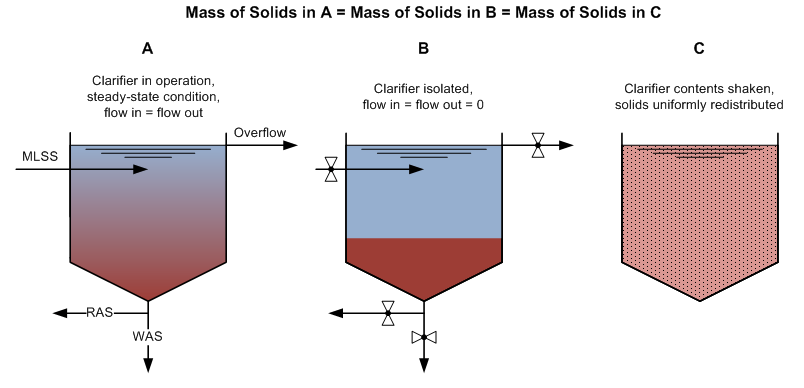If you were to stop all flow in and out of the clarifier you would have Scenario B. In Scenario B, after some period of time, you will have settled all of the solids and a sludge blanket of some depth will have accumulated on the bottom of the clarifier. The water above the sludge blanket will be clear and relatively free of solids. After all the solids have settled in Scenario B, the concentration at the bottom of the tank would be equal to the RAS concentration.

Given Scenario B, if you were to theoretically shake the tank up so that the solids in the sludge blanket are completely and uniformly redistributed throughout the clarifier, as depicted in Scenario C, the total suspended solids of this concentration would be equal to the MLSS concentration which was the original source of flow into the clarifier. So it is reasonable to use the MLSS concentration and the entire volume of the clarifier when calculating the total solids in the biological treatment system as part of the MCRT formula. This is certainly the easiest way to include the solids in the clarifier when calculating the MCRT because it does not require any additional sampling.

This is the method (4) I used when I compared SRT to MCRT in Table No. 1.

For a guide I refer you to Table No. 2 which provides SRT ranges for achieving specific treatment goals. This table is from Metcalf & Eddy, 2003.

Table No. 2: SRT Ranges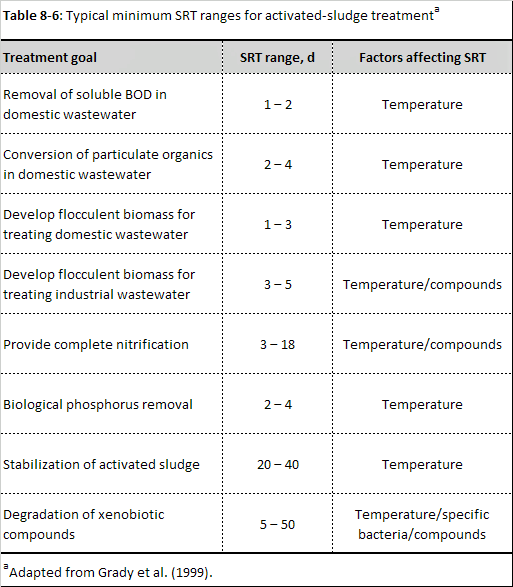Recall from the distribution fit in Figure No. 3 the mean SRT for an industrial wastewater plant treating complex wastewater was 13 days with a maximum value of 28 days.

Also, from Table No. 2, the stabilization of activated sludge with an SRT range of 20 to 40 days is in reference to an aerobic digester.

Xenobiotic: relating to or denoting a substance, typically a synthetic chemical, that is foreign to the body or to an ecological system.

Tags:

bottom of page